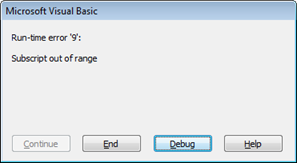# xlf QandA series

## How to determine if a worksheet exists in a workbook

### QUESTION

I WANT TO INSERT A SERIES OF NEW WORKSHEETS WITH A MACRO. HOW CAN I TELL IF THE WORKSHEET NAME ALREADY EXISTS IN THE WORKBOOK?

###Answer

The ExistsSheet procedures developed in this page use the Sheets.Name property to find if a worksheet or chartsheet name already exists in the workbook. If the name cannot be found, then the sheet does not exist.

A Worksheet or ChartSheet object is a member of the Sheets collection. To return a single sheet, use Sheets(index), where index is the sheet name (section 1 - code 1) or index number (section 2 - code 3).

### 1. Sheets(InName).Name = Empty

Suppose that Sheet1 is a tab name in the workbook, then the expression Sheets("Sheet1").Name returns the name Sheet1, if not, it returns a Run-time error '9', Subscript out of range error as shown in figure 1.Fig 1: The On Error Resume Next statement - stops this Run time error message from displaying

In code 1, the custom function ExistsSheet uses an On Error Resume Next statement to disable the error-handling routine and suppress the run-time error message. When this is done, the Sheets.Name statement in line 3 of the code returns Empty in the case when the name does not exist. If the statement Sheets(InName).Name = Empty is TRUE, then the sheet does not exist and ExitsSheet returns FALSE. If the statement is FALSE, that is, not empty, then the sheet does exist and ExistsSheet returns TRUE.

Code 1: Function ExistsSheet returns TRUE if InName exists and FALSE if not
Function ExistsSheet(InName As String) As Boolean
On Error Resume Next
If Sheets(InName).Name = Empty Then
ExistsSheet = False
Else
ExistsSheet = True
End If
On Error GoTo 0
End Function


The TestExistsSheet procedure in the code 2 frame demonstrates the ExistsSheet function. You can change the TestSheet variable in lines 5 and 6 to try different sheet names.

Code 2: A macro to test the ExistsSheet function
Sub TestExistsSheet()
Dim TestSheet(1 To 2) As String
Dim i As Long
' Workbook contains only Sheet1, Sheet2, and Sheet3
TestSheet(1) = "Sheet3"
TestSheet(2) = "Sheet4"
For i = 1 To 2
If ExistsSheet(TestSheet(i)) = True Then
MsgBox TestSheet(i) + " exists."
Else
MsgBox TestSheet(i) + " does NOT exist", vbInformation, "xlf ExistsSheet"
End If
Next i
End Sub


The output is sent to two consecutive message boxes.

In VBA the concatenation operator is the & character, the same as Excel, but VBA also allows use of the + operator for concatenation of text strings, as shown in lines 9 and 11 of code 2.

### 2. Sheets(i).Name = InName

By using the index number, rather than the name, we avoid triggering Run-time error '9'. The For...Next loop in code 3 only calls the Name property of existing sheets.

Code 3: Function ExistsSheet2 returns TRUE if InName exists and FALSE if not
Function ExistsSheet2(InName As String) As Boolean
For i = 1 To Sheets.Count
If Sheets(i).Name = InName Then
ExistsSheet2 = True
Exit For
Else
ExistsSheet2 = False
End If
Next i
End Function


• This example was developed in Excel 2013 Pro 64 bit.
• First published: 30th July 2015
• Revised: Saturday 23rd of June 2018 - 07:10 PM, [Australian Eastern Standard Time (EST)]